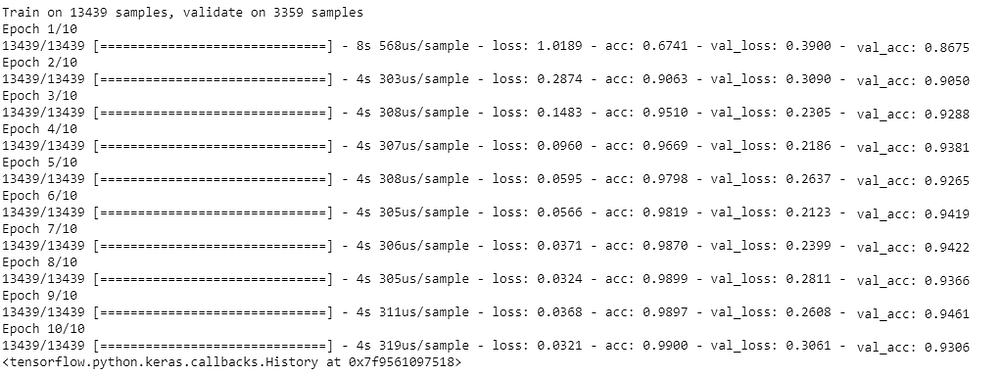# Convolutional Neural Network on AHCD dataset

Source: Deep Learning on Medium# Convolutional Neural Network on AHCD dataset

Convolutional Neural Networks ( CNNs ) are one of the most powerfull and used supervised learning techniques in deep learning mainly in image classification.

Handwritten character recognition systems in Arabic are very rare and
they face a lot of difficulties, including the unlimited variation of human writing and very large datasets.

In this article we will train a simple Convolutional Neural Network for Arabic Handwritten Characters Dataset ( AHCD ) to classify arabic letters. Befor we dive into the making process of the convnet I prefere to give you a breaf introduction to CNNs if you don’t know what it is and why we use it.

# What are Convolutional neural networks :

Convolutional neural networks are very similar to regular neural networks ( multy layer perceptron MLPs ), they both are made up of neurons having learnable parameters and uses a loss function to adjust them. The main difference is that ConvNets make the assumption that the inputs are images explicitly, this reduces vastly the amount of parameters and learn much faster . ConvNets are really good at extracting features, they can capture and learn features from an image at different levels.

## ConvNets VS. MLP :

In multy layer perceptrons, every element of a previous layer is connected to every element of the next layer. So for example if you have a grayscaled image of 28×28 pixels, you will end up with 28x28x1 (784) neurons which can be manageable. But in the case of big rgb images or high resolution images, you will likely end up with thousands of neurons in the input layer which is without doubt non practicle therefor MLPs are not scalable when it comes to image classification. ConvNets can solve this problem as they are weight sharing, if you have a one layer CNN with 10 3×3 filters the number of parameters would be 3x3x10+10 = 100 parameters ( +10 being the number of biases ). Now if we take the previous 784 input neurons MLP, having one hidden layer of 200 neurons, the number of parameters would be 784×200+1=156801. Clearly CNNs are a lot less complex .

# Building the CNN for AHCD :

## preparing the data :

Ahcd dataset is composed of 16,800 characters written by 60 participants. The database is partitioned into two sets: a training set (13,440 characters to 480 images per class) and a test set (3,360 characters to 120 images per class). The caracters are labeled from “ا “ to “ي “ ( aliph to ya ). Each Image is of size 32 by 32 and grayscaled. You can download the dataset from here.

`#Loading the datasetx_train = pd.read_csv(‘csvTrainImages 13440x1024.csv’)y_train = pd.read_csv(‘csvTrainLabel 13440x1.csv’)x_test = pd.read_csv(‘csvTestImages 3360x1024.csv’)y_test = pd.read_csv(‘csvTestLabel 3360x1.csv’)`
`x_train = np.asarray(x_train).reshape(x_train.shape,32,32,1)x_test = np.asarray(x_test).reshape(x_test.shape,32,32,1)`